This is a page containing supporting movies for the paper: "Nonlinear conduction via solitons in a topological mechanical insulator", by Bryan Gin-ge Chen, Nitin Upadhyaya, and Vincenzo Vitelli at the Instituut-Lorentz for Theoretical Physics at Leiden University.

(S1) Bulk Rigidity, Exponentially Localized Mode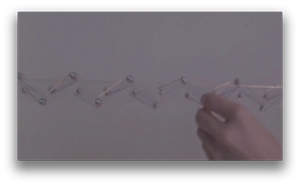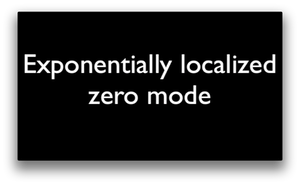(S2) Conductor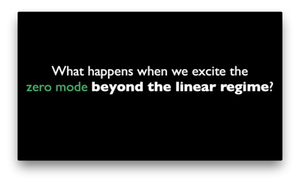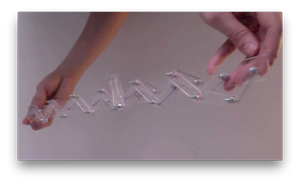This movie shows how the exponentially localized mode integrates into a moving domain wall in the model chain.
(S3) Flipper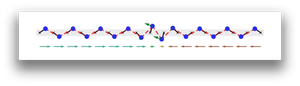This movie shows a simulation (Newtonian dynamics) of the full cycle of a (non-wobbling) flipper soliton in a chain of 17 rotors. The geometrical parameters of the chain are a = 1, r = 0.5 and \bar\theta = 0.97. The other model parameters were k_e = 1000, M = 1, and v_0 = 0.08. The green arrows on each rotor are proportional to the angular velocity, and the red and blue arrows underneath depict the x-projections of the rotors, showing the domain wall nature of the soliton.
(S4) Wobbling flipper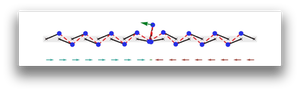This movie shows a simulation of the full cycle of a wobbling flipper soliton in a chain of 17 rotors. The geometrical parameters of the chain are a = 1, r = 1.1 and \bar\theta = 1.18. The other model parameters were k_e = 1000, M = 1, and v_0 = 0.04. The green arrows on each rotor are proportional to the angular velocity, and the red and blue arrows underneath depict the x-projections of the rotors, showing the domain wall nature of the soliton.
(S5) Spinner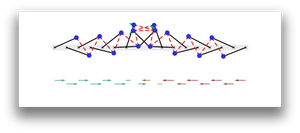This movie shows a simulation of the full cycle of a spinner soliton in a chain of 17 rotors. The geometrical parameters of the chain are a = 1, r = 2 and \bar\theta = 1.18. The other model parameters were k_e = 1000, M = 1, and v_0 = 0.04. The green arrows on each rotor are proportional to the angular velocity, and the red and blue arrows underneath depict the x-projections of the rotors, showing the domain wall nature of the soliton. We offset the x-projections of the odd and even-numbered sites to emphasize that the angles of odd rotors and even rotors converge separately to smooth functions differing by a constant.
(S6) Flipper unit cell configuration space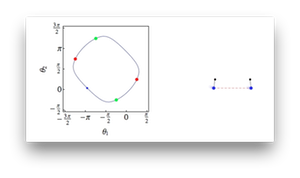This movie shows a unit cell of a chain in the (non-wobbling) flipper phase and its allowed motion in the configuration space spanned by the two rotor angles. The geometrical parameters of the chain are a = 1, r = 0.25 and \bar\theta = 0.79.
(S7) Wobbling flipper unit cell configuration space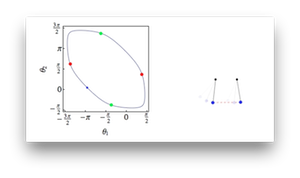This movie shows a unit cell of a chain in the wobbling flipper phase and its allowed motion in the configuration space spanned by the two rotor angles. The geometrical parameters of the chain are a = 1, r = 1.1 and \bar\theta = 1.18.
(S8) Spinner unit cell configuration space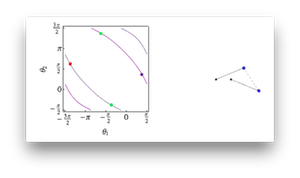This movie shows a unit cell of a chain in the (non-wobbling) flipper phase and its allowed motion in the configuration space spanned by the two rotor angles. The geometrical parameters of the chain are a = 1, r = 2 and \bar\theta = 1.18.
(S9) Spinner Realization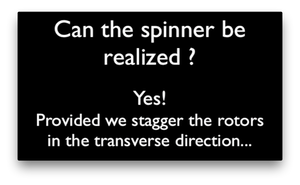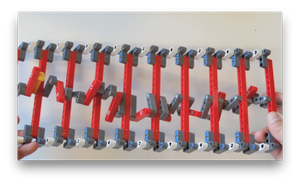This movie shows a model of the chain of rotors made out of LEGO in the spinner phase.